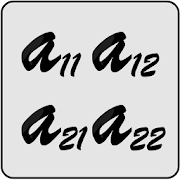Matrix Calculator

Fully detailed matrix calculator for matrix operations

3.76 EDUCATION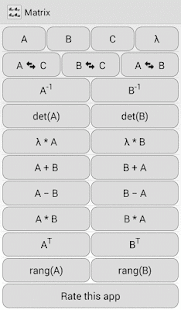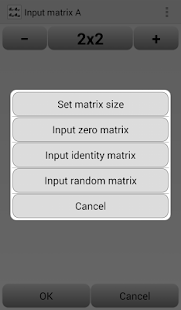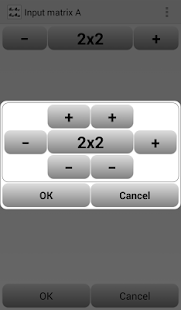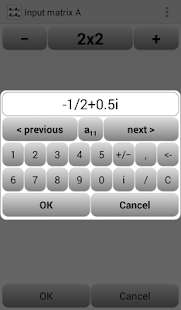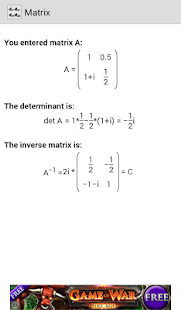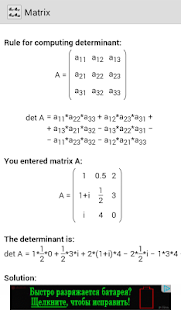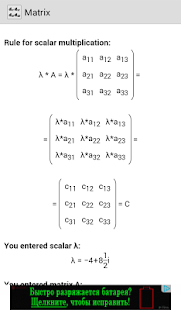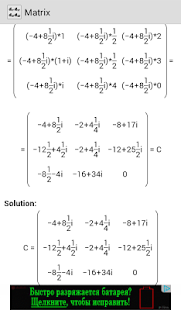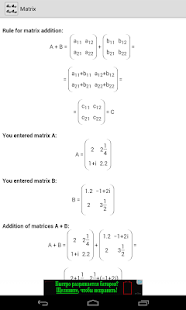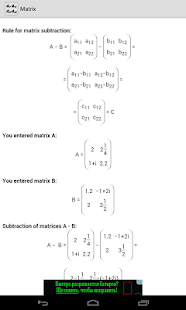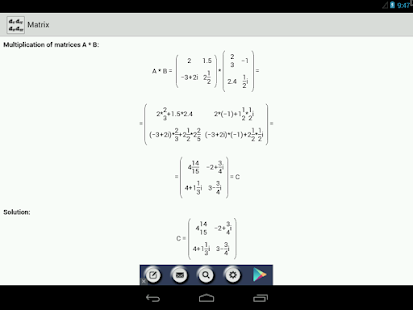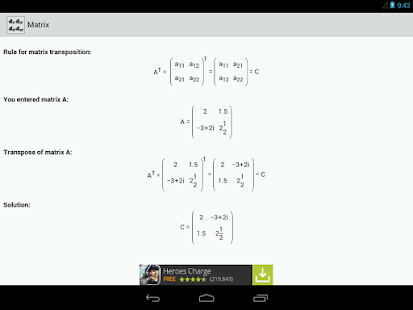This application is absolutely free mathematical calculator.
Its main task – calculate mathematical matrices.

Supported matrix operations:
- Matrix Inverse.
- Matrix Determinant.
- Matrix Scalar multiplication.
- Matrix Subtraction.
- Matrix Multiplication.
- Matrix Transposition.

The application can work with:
- Integers (-2, -1, 0, 1, 2 etc.).
- Decimal fractions (1.5, 3.14 etc.).
- Common fractions (1/2, 3/4, 7/3 etc.).
- Complex numbers (i, 1+i, 1/2-2i, 0.5+2/3i etc.).

- Bug fixes.

Details and Recent Ratings

Size: 0 MB
Version: 3.05 by Alexander Skokov
Updated: 12 June 2015 (2417 days ago)
Released: 30 March 2014
Installations: more than 100 000
5 Stars: 1779
4 Stars: 483
3 Stars: 219
2 Stars: 153
1 Star: 659

Similar apps

Android Game Categories
Android App Categories